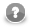### Assignment Operator

Assignment operator assigns a value of expression on the right side of the operator to a variable on the left side of the operator.

`int i = 5;`

#### Compound Operators

Compound operators allow you to use a variable as an accumulator.

Since CloverETL 4.1.0-M1, CTL2 supports the following compound assignment operators: `+=` (addition, string concatenation, list concatenation and map union), `-=` (subtraction), `*=` (multiplication), `/=` (division), and `%=` (modulus).

If the original value of the left-hand side variable is `null`, the default value for the target type (0, empty string, empty list, empty map) is used for the evaluation instead. See variables `ns` and `ns2` in the example below.

Example 66.17. Compound assignment operators

```  integer i = 5;
i += 4; // i == 9

integer ni = null;
ni += 5; // ni == 5

string s = "hello ";
s += "world "; // s == "hello world "
s += 123; // s == "hello world 123"

string ns = null;
ns += "hello"; // ns == "hello"

string ns2 = null;
ns2 = ns2 + "hello"; // ns2 == "nullhello"

integer[] list1 = [1, 2, 3];
integer[] list2 = [4, 5];
list1 += list2; // list1 == [1, 2, 3, 4, 5]

map[string, integer] map1;
map1["1"] = 1;
map1["2"] = 2;
map[string, integer] map2;
map2["2"] = 22;
map2["3"] = 3;
map1 += map2; // map1: "1"->1, "2"->22, "3"->3

long l = 10L;
l -= 4; // l == 6L;

decimal d = 12.34D;
d *= 2; // d == 24.68D;

number n = 6.15;
n /= 1.5; // n ~ 4.1

long r = 27;
r %= 10; // r == 7L```

NoteCTL2 does not perform any counter-intuitive conversion of the right operand of `+=`. If you need to add double to integer, you should convert it explicitly: ```integer i = 3; i += double2integer(1.0);``` It works with `-=`, `*=`, `/=` and `%=` as well.

As of CloverETL 3.3, the = operator does not just pass object references, but performs a deep copy of values. That is of course more demanding in terms of performance. Deep copy is only performed for mutable data types, i.e. lists, maps, records and dates. Other types are considered immutable, as CTL2 does not provide any means of changing the state of an existing object (even though the object is mutable in Java). Therefore it is safe to pass a reference instead of copying the value. Note that this assumption may not be valid for custom CTL2 function libraries.

Example 66.18. Modification of a copied list, map and record

```  integer[] list1 = [1, 2, 3];
integer[] list2;
list2 = list1;

list1.clear(); //  only list1 is cleared (older implementation: list2 was cleared, too)

map[string, integer] map1;
map1["1"] = 1;
map1["2"] = 2;
map[string, integer] map2;
map2 = map1;

map1.clear(); //  only map1 is cleared (older implementation: map2 was cleared, too)